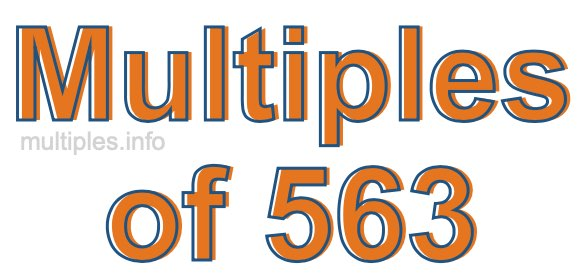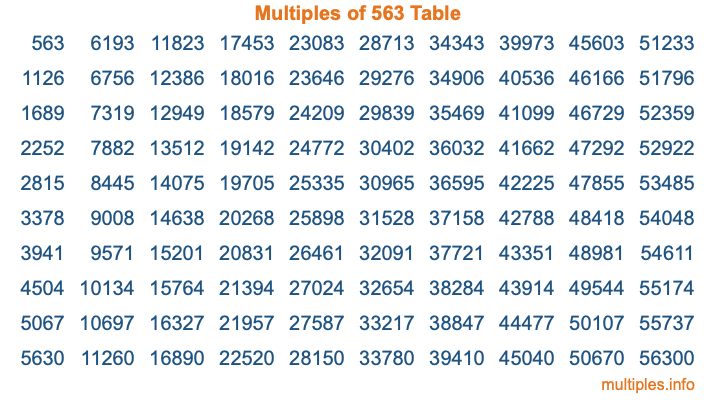Multiples of 563Welcome to the Multiples of 563 page. Here we will first teach you everything you will ever need to know about the multiples of 563, and then give you a study guide summary of everything we taught you to make sure you remember it all. Use this page to look up facts and learn information about the multiples of 563. This page will make you a multiples of five hundred sixty-three expert!

Definition of Multiples of 563
Multiples of 563 are all the numbers that when divided by 563 equal an integer. Each of the multiples of 563 are called a multiple. A multiple of 563 is created by multiplying 563 by an integer.

Therefore, to create a list of multiples of 563, you start with 1 multiplied by 563, then 2 multiplied by 563, then 3 multiplied by 563, and so on for as long as you want. Thus, the list of the first five multiples of 563 is 563, 1126, 1689, 2252, and 2815. To see a larger list of multiples of 563, see the printable image of Multiples of 563 further down on this page. We also have a category where you can choose any nth multiple of 563.

Multiples of 563 Checker
The Multiples of 563 Checker below checks to see if any number of your choice is a multiple of 563. In other words, it checks to see if there is any number (integer) that when multiplied by 563 will equal your number. To do that, we divide your number by 563. If the the quotient is an integer, then your number is a multiple of 563.

Is  a multiple of 563?

Least Common Multiple of 563 and ...
A Least Common Multiple (LCM) is the lowest multiple that two or more numbers have in common. This is also called the smallest common multiple or lowest common multiple and is useful to know when you are adding our subtracting fractions. Enter one or more numbers below (563 is already entered) to find the LCM.

Check out our LCM Calculator if you need more details about the Least Common Multiple or if you need the LCM for different numbers for adding and subtraction fractions.

nth Multiple of 563
As we stated above, 563 is the first multiple of 563, 1126 is the second multiple of 563, 1689 is the third multiple of 563, and so on. Enter a number below to find the nth multiple of 563.

th multiple of 563

Multiples of 563 vs Factors of 563
563 is a multiple of 563 and a factor of 563, but that is where the similarities end. All postive multiples of 563 are 563 or greater than 563. All positive factors of 563 are 563 or less than 563.

Below is the beginning list of multiples of 563 and the factors of 563 so you can compare:

Multiples of 563: 563, 1126, 1689, 2252, 2815, etc.

Factors of 563: 1, 563

As you can see, the multiples of 563 are all the numbers that you can divide by 563 to get a whole number. The factors of 563, on the other hand, are all the whole numbers that you can multiply by another whole number to get 563.

It's also interesting to note that if a number (x) is a factor of 563, then 563 will also be a multiple of that number (x).

Multiples of 563 vs Divisors of 563
The divisors of 563 are all the integers that 563 can be divided by evenly. Below is a list of the divisors of 563.

Divisors of 563: 1, 563

The interesting thing to note here is that if you take any multiple of 563 and divide it by a divisor of 563, you will see that the quotient is an integer.

Multiples of 563 Table
Below is an image of the first 100 multiples of 563 in a table. The table is in chronological order, column by column. The first column has the first ten multiples of 563, the second column has the next ten multiples of 563, and so on.The Multiples of 563 Table is also referred to as the 563 Times Table or Times Table of 563. You are welcome to print out our table for your studies.

Negative Multiples of 563
Although not often discussed or needed in math, it is worth mentioning that you can make a list of negative multiples of 563 by multiplying 563 by -1, then by -2, then by -3, and so on, to get the following list of negative multiples of 563:

-563, -1126, -1689, -2252, -2815, etc.

Multiples of 563 Summary
Below is a summary of important Multiples of 563 facts that we have discussed on this page. To retain the knowledge on this page, we recommend that you read through the summary and explain to yourself or a study partner why they hold true.

There are an infinite number of multiples of 563.

A multiple of 563 divided by 563 will equal a whole number.

563 divided by a factor of 563 equals a divisor of 563.

The nth multiple of 563 is n times 563.

The largest factor of 563 is equal to the first positive multiple of 563.

563 is a multiple of every factor of 563.

563 is a multiple of 563.

A multiple of 563 divided by a divisor of 563 equals an integer.

563 divided by a divisor of 563 equals a factor of 563.

Any integer times 563 will equal a multiple of 563.

Multiples of a Number
Here you can get the multiples of another number, all with the same attention to detail as we did for multiples of 563 on this page.

Multiples of
Multiples of 564
Did you find our page about multiples of five hundred sixty-three educational? Do you want more knowledge? Check out the multiples of the next number on our list!